Next: Potential 4-Vector Up: Relativity and Electromagnetism Previous: 4-Velocity and 4-Acceleration

# Current Density 4-Vector

Let us now consider the laws of electromagnetism. We wish to demonstrate that these laws are compatible with the relativity principle. In order to achieve this, it is necessary for us to make an assumption about the transformation properties of electric charge. The assumption that we shall make, which is well substantiated experimentally, is that charge, unlike mass, is invariant. That is, the charge carried by a given particle has the same measure in all inertial frames. In particular, the charge carried by a particle does not vary with the particle's velocity.

Let us suppose, following Lorentz, that all charge is made up of elementary particles, each carrying the invariant amount. Suppose that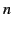is the number density of such charges at some given point and time, moving with velocity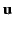, as observed in a frame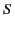. Let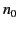be the number density of charges in the frame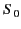in which the charges are momentarily at rest. As is well known, a volume of measure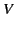inhas measure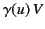in(because of length contraction). Because observers in both frames must agree on how many particles are contained in the volume, and, hence, on how much charge it contains, it follows that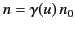. If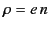andare the charge densities inand, respectively, then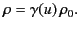(1726)

The quantity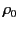is called the proper density, and is obviously Lorentz invariant.

Suppose that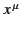are the coordinates of the moving charge in. The current density 4-vector is constructed as follows: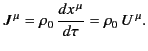(1727)

Thus,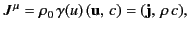(1728)

where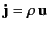is the current density 3-vector. Clearly, charge density and current density transform as the time-like and space-like components of the same 4-vector.

Consider the invariant 4-divergence of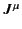: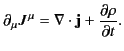(1729)

We know that one of the caveats of Maxwell's equations is the charge conservation law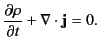(1730)

It is clear that this expression can be rewritten in the manifestly Lorentz invariant form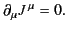(1731)

This equation tells us that there are no net sources or sinks of electric charge in nature: that is, electric charge is neither created nor destroyed.Next: Potential 4-Vector Up: Relativity and Electromagnetism Previous: 4-Velocity and 4-Acceleration
Richard Fitzpatrick 2014-06-27